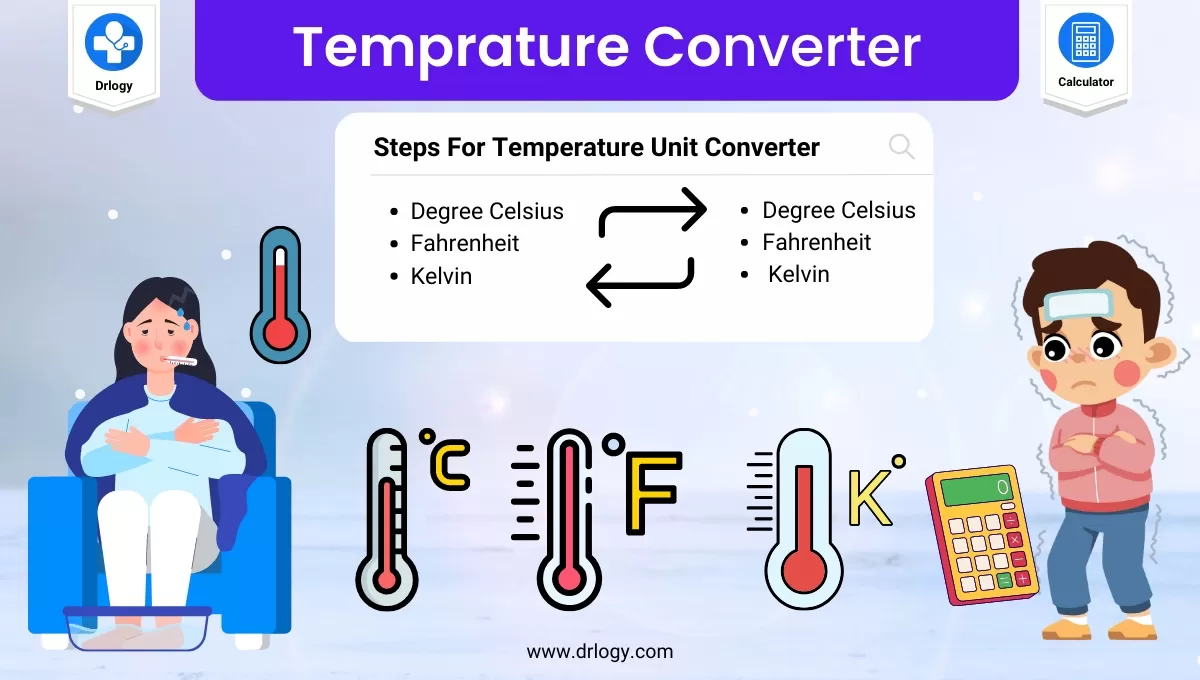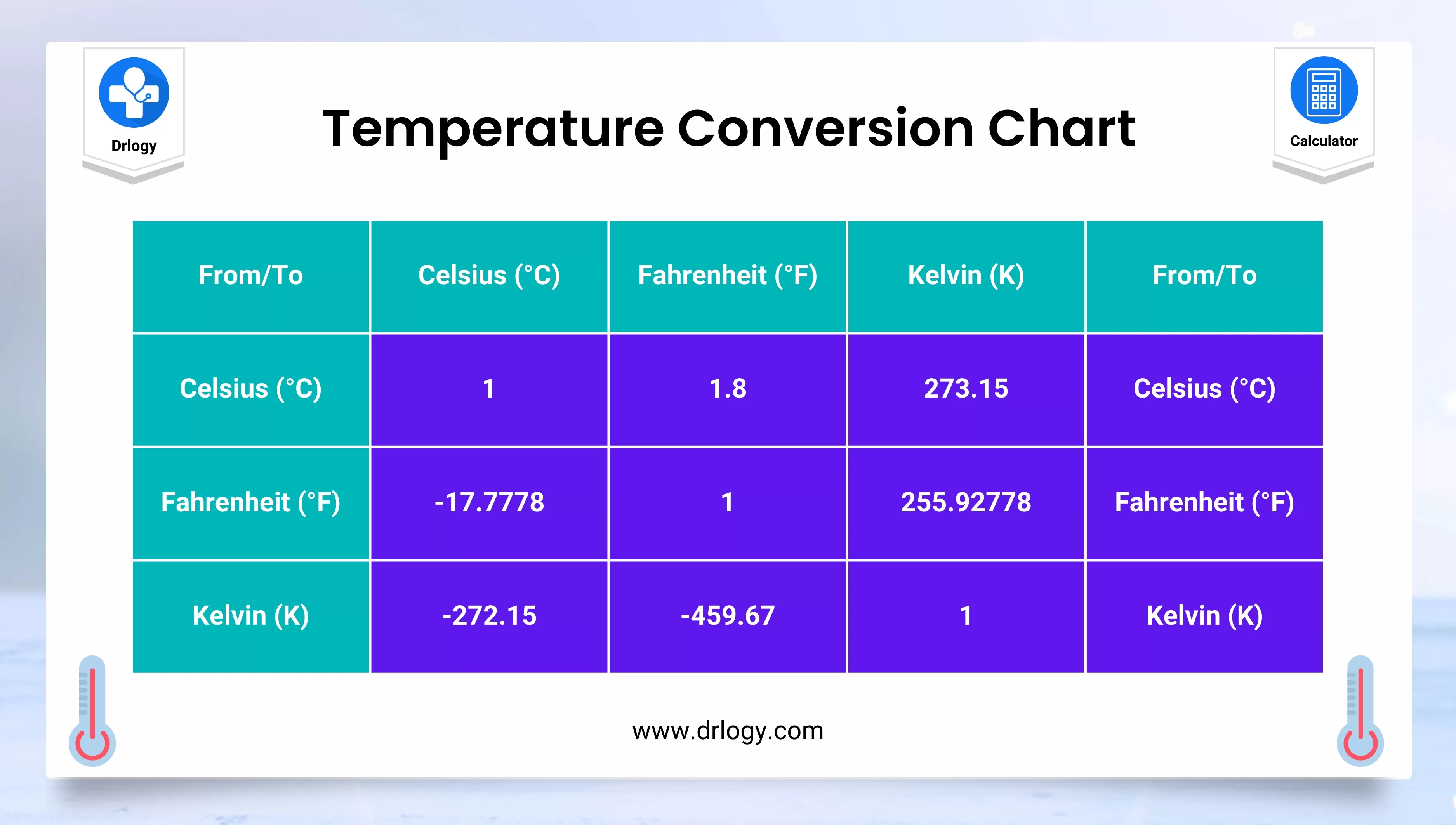• Health
• Pet
• Unit Conversion
• Medical# Temperature Converter Calculator For Unit Conversion

## Temperature Converter Calculator

keyboard_arrow_down
=
keyboard_arrow_down

## Temperature Converter

• Converting temperatures between Celsius, Fahrenheit, and Kelvin can be a little tricky.
• Fortunately, there are temperature converter calculators that make it easy.
• Temperature Converter Calculator will show you how to use a temperature converter calculator to convert temperatures between the three most commonly used temperature scales.

## Steps For Temperature Converter Calculator

A temperature converter calculator can be used to convert temperatures between different temperature scales, such as Celsius, Fahrenheit, and Kelvin. Here's how you can use it:

1. Decide which temperature scale you want to convert from and which one you want to convert to. For example, you may want to convert a temperature from Celsius to Fahrenheit, or from Kelvin to Celsius.
2. Open a temperature converter calculator in your web browser or on your smartphone. There are many temperature converter calculators available online, both as websites and mobile apps.
3. Enter the temperature value that you want to convert. For example, if you want to convert 20 degrees Celsius to Fahrenheit, enter the value "20" in the Celsius input box.
4. Select the temperature scale that corresponds to the value you entered. For example, if you entered "20" in the Celsius input box, select "Celsius" as the input scale.
5. Select the temperature scale that you want to convert to. For example, if you want to convert from Celsius to Fahrenheit, select "Fahrenheit" as the output scale.
6. Click the "Convert" button or hit enter on your keyboard. The temperature converter calculator will automatically display the converted temperature value in the output box. In our example, the converted value would be displayed as "68" in the Fahrenheit output box.
7. If you want to convert another temperature, simply repeat the process from step 3.

Temperature converter calculators are easy to use and can save you a lot of time and hassle when you need to convert temperatures. They are particularly useful for scientific or engineering applications where precise temperature conversions are necessary.

## Temperature Converter Formula

Here is the 3 most common Temperature converter calculator formula for basic temperature conversion.

The temperature converter calculator will show the steps to solve the conversion equation for:

• Celsius to Fahrenheit
• Celsius to Kelvin
• Fahrenheit to Celsius
• Fahrenheit to Kelvin
• Kelvin to Celsius
• Kelvin to Fahrenheit

### Celsius Temperature Conversion Formula

 Celsius to Fahrenheit Conversion °F = °C × 9/5 + 32 Celsius to Kelvin Conversion K = °C + 273.15 Celsius to Rankine Conversion °R = °C × 9/5 + 491.67

### Fahrenheit Temperature Conversion Formula

 Fahrenheit to Celsius Conversion °C = (°F − 32) × 5/9 Fahrenheit to Kelvin Conversion K = (°F + 459.67) × 5/9 Fahrenheit to Rankine Conversion °R = °F + 459.6

### Kelvin Temperature Conversion Formula

 Kelvin to Celsius Conversion °C = K − 273.15 Kelvin to Fahrenheit Conversion °F = K × 9/5 − 459.67 Kelvin to Rankine Conversion °R = K × 9/5

## Temperature Converter CalculatorBody: Before we dive into the conversions, let's first understand the three temperature scales:

### Celsius

• This is the most commonly used temperature scale worldwide.
• It is based on the freezing point (0°C) and boiling point (100°C) of water at sea level.

### Fahrenheit

• This temperature scale is used primarily in the United States.
• It is based on the freezing point (32°F) and boiling point (212°F) of water at sea level.

### Kelvin

• This temperature scale is based on the absolute zero point (0 K), which is the point at which all molecular motion stops.
• It is used primarily in scientific and engineering applications.

## Degrees Temperature Converter

Now that we understand the three temperature scales, let's learn how to convert between them using a temperature converter calculator.

### Celsius to Fahrenheit

• To convert Celsius to Fahrenheit, simply enter the Celsius temperature into the converter and click the "Convert" button.
• The converter will automatically display the equivalent Fahrenheit temperature.

### Fahrenheit to Celsius

• To convert Fahrenheit to Celsius, enter the Fahrenheit temperature into the converter and click the "Convert" button.
• The converter will automatically display the equivalent Celsius temperature.

### Celsius to Kelvin

• To convert Celsius to Kelvin, enter the Celsius temperature into the converter and click the "Convert" button.
• The converter will automatically display the equivalent Kelvin temperature.

### Kelvin to Celsius

• To convert Kelvin to Celsius, enter the Kelvin temperature into the converter and click the "Convert" button.
• The converter will automatically display the equivalent Celsius temperature.

### Fahrenheit to Kelvin

• To convert Fahrenheit to Kelvin, first convert the Fahrenheit temperature to Celsius using the converter.
• Then enter the Celsius temperature into the converter and click the "Convert" button.
• The converter will automatically display the equivalent Kelvin temperature.

### Kelvin to Fahrenheit

• To convert Kelvin to Fahrenheit, first convert the Kelvin temperature to Celsius using the converter.
• Then enter the Celsius temperature into the converter and click the "Convert" button.
• The converter will automatically display the equivalent Fahrenheit temperature.

Converting temperatures between Celsius, Fahrenheit, and Kelvin can be a little tricky, but with a temperature converter calculator, it's a breeze. Simply enter the temperature you want to convert and click the "Convert" button. The converter will do the rest. Happy converting!

## Temperature Converter Calculator Benefits

There are several advantages and benefits to using a temperature converter calculator:

1. Accurate conversions: Temperature converter calculators provide accurate conversions between different temperature scales. This is particularly important when precise temperature measurements are required, such as in scientific or engineering applications.
2. Time-saving: Converting temperatures manually can be time-consuming and prone to errors. A temperature converter calculator eliminates the need for manual calculations and provides quick and easy conversions in just a few clicks.
3. Convenience: Temperature converter calculators are widely available online and can be accessed from any device with an internet connection. This makes them convenient to use when you need to convert temperatures on the go.
4. Easy to use: Temperature converter calculators are user-friendly and require no special training or expertise to use. Simply enter the temperature you want to convert and select the temperature scale you want to convert to, and the calculator will do the rest.
5. Multiple conversions: Many temperature converter calculators allow you to convert between multiple temperature scales, such as Celsius, Fahrenheit, and Kelvin. This makes them versatile tools that can be used for a wide range of applications.
6. Free accessibility: Many temperature converter calculators are available online for free, making them an accessible tool for anyone who needs to convert temperatures.

Summary

Overall, a temperature converter calculator is a valuable tool that provides accurate, convenient, and time-saving conversions between different temperature scales. Check more unit conversion calculators like this to solve your daily problems on Drlogy Calculator to get exact solution.

Reference

• Conversion of scales of temperature - Wikipedia .
• Scale of temperature .
favorite_border 15890 Likes

## Temperature Converter Calculator FAQ

### How do you calculate F to C?

To convert a temperature from Fahrenheit (°F) to Celsius (°C), use the following formula:

(°F - 32) x 5/9 = °C

Here's an example:

Convert 68°F to Celsius.

(68 - 32) x 5/9 = 20°C

Therefore, 68°F is equal to 20°C.

### How to convert quick C to F?

To convert a temperature from Celsius (°C) to Fahrenheit (°F) quickly, you can use the following shortcut formula:

°C x 1.8 + 32 = °F

Here's an example:

Convert 20°C to Fahrenheit.

20 x 1.8 + 32 = 68°F

Therefore, 20°C is equal to 68°F using this shortcut formula.

Note that while this shortcut formula is quick and easy to use, it may not provide as precise results as the full formula. If you need a more accurate conversion, you should use the full formula, which is:

(°C x 9/5) + 32 = °F

### What temp is C to F?

To convert a temperature from Celsius (°C) to Fahrenheit (°F), use the following formula:

(°C x 9/5) + 32 = °F

This formula involves multiplying the temperature in Celsius by 9/5 and then adding 32 to the result to get the temperature in Fahrenheit.

For example, to convert 20°C to Fahrenheit:

(20 x 9/5) + 32 = 68°F

Therefore, 20°C is equal to 68°F.

## Financial Calculator

• Home >
• Calculator >
• Temperature Converter Calculator For Unit Conversion
Drlogy®
The Power To Health